World Journal of Applied Chemistry
Volume 2, Issue 1, February 2017, Pages: 1-6

Molecular Dynamics Calculation on Mechanical Properties and Binding Energy of JOB-9003 Explosive at Different Temperatures

Guiyun Hang*, Wenli Yu, Tao Wang, Zhen Li

Department of Nuclear Engineering, Xi’an Research Institute of High-Tech, Xi’an, China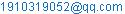(Guiyun Hang)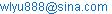(Wenli Yu)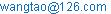(Tao Wang)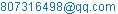(Zhen Li)

*Corresponding author

Guiyun Hang, Wenli Yu, Tao Wang, Zhen Li. Molecular Dynamics Calculation on Mechanical Properties and Binding Energy of JOB-9003 Explosive at Different Temperatures. World Journal of Applied Chemistry. Vol. 2, No. 1, 2017, pp. 1-6. doi: 10.11648/j.wjac.20170201.11

Received: November 1, 2016; Accepted: December 29, 2016; Published: January 20, 2017

Abstract: To research the effect of temperature on mechanical properties and binding energy of JOB-9003 explosive, the crystal model of JOB-9003 explosive was established by Materials Studio (MS). Molecular dynamics simulation was applied to investigate the mechanical properties and binding energy of explosive by COMPASS (condensed-phase optimized molecular potentials for atomistic simulation studies) force field at different temperatures (195 K, 245 K, 295 K, 345 K, 395 K, 445 K). The results show that with the increasing of temperature, the mechanical properties (tensile modulus, shear modulus, bulk modulus and Cauchy pressure) of JOB-9003 explosive decrease gradually, which indicates that the rigidity and hardness of the explosive becomes worse, while the toughness and plastic property becomes better and it agrees with theoretical analysis result, thus illustrating that MD simulation can be used to predict the mechanical properties of JOB-9003 explosive. The results of binding energy show that the binding energy decreases with the increasing of temperature, thus indicating that the stability of explosive becomes worse. In other words, the increasing of temperature has a negative effect on mechanical properties and binding energy of JOB-9003 explosive. This paper could provide some theoretical references and technological support for the comprehensive assessment of mechanical properties and stability of explosives.

Keywords: Physical Chemistry, JOB-9003 Explosive, Mechanical Properties, Binding Energy, Materials Studio, Molecular Dynamics

Contents

1. Introduction

As well known, JOB-9003 explosive mainly consists of octahydro-1, 3, 5, 7-tetranitro-1, 3, 5, 7- tetrazocine (HMX) and it has notable merits such as high energy density, excellent detonation performance, improved mechanical properties and so on. Therefore, JOB-9003 explosive is one of the most important polymer-bonded explosives (PBXs) and it has been widely used in both military and industry areas for a long time.

During the production, storage and transportation process, the external temperature may be changed and it would influence the mechanical properties and stability of JOB-9003 explosive. Influenced by this, the comprehensive performance and safety of the weapons will be affected. Therefore, it is of great significance and importance to research the effect of temperature on mechanical properties and binding energy of explosive.

To calculate the mechanical properties and binding energy of JOB-9003 explosive, the mainly used software is Materials Studio (MS)  and molecular dynamics (MD) simulation is the commonly used method. At present, MD method has played an important role in investigating the high energy density materials and it blazes a new way for the development of new energetic materials. Xiufang Ma et al  researched the structure and properties of HMX based four components composite material by molecular dynamics method. Fang Chen et al  investigated the effects of polymer concentration and temperature on mechanical properties and binding energies of ε-CL-20-based polymer bonded explosives (PBXs). Dongxu Wang et al  investigated the mechanical behavior of 2, 4, 6, 8, 10, 12-hexanitro-2, 4, 6, 8, 10, 12-hexaazaisowurtzitane (ε-HNIW)/F2311 inter-face structure. Dongmei Liu et al  explored and compared the progressive changes of structure, energetic and mechanical properties of cyclotrimethylene trinitramine (RDX) and cyclotetramethylene tetranitra- mine (HMX) crystals at different temperatures by molecular dynamics simulation. What’s more, a lot of researchers have used MS to conduct investigations on molecular structures, mechanical properties, sensitivity, stability, binding energy and detonation performance of energetic materials and high energy density explosives and a great deal of achievements have been got [6-14].

In this article, crystal model of JOB-9003 explosive was established in MS and MD method was applied to calculate the mechanical properties and binding energy of explosive at different temperatures. The main aim of the research was to investigate the effect of temperature on mechanical properties and binding energy of explosive. The results could provide some references for the comprehensive performance assessment of explosives.

2. Calculation Model and Method

2.1. Single Molecular Model

JOB-9003 explosive consists of octahydro-1, 3, 5, 7- tetranitro-1, 3, 5, 7-tetrazocine (HMX), 1, 3, 5-triamino- 2, 4, 6-trinitrobenzene (TATB), fluorine rubber (F2311) and paran wax (PW), F2311 is copolymerized from vinylidenedifluoride and chlorotrifluoroethylene with the molar ratio of 1:1 and F or H atoms are used to saturate the end groups according to their types. The mass ratio of HMX, TATB, F2311 and PW in JOB-9003 explosive is 87%, 7%, 4.2% and 1.8%, respectively.

The molecular models of HMX, TATB, F2311 and PW are shown in Figure. 1 to Figure. 4, respectively, the crystal polymorph of HMX is chosen as β-HMX, because among the four known polymorphic forms, β-HMX is the most stable phase at room temperature [15-16]. The molecular formula of F2311 and PW is determined based on the mass ratio in JOB-9003 explosive. By calculation, we can confirm that the molecular formula of F2311 is C20H10F26Cl6 and it is C29H60 of PW.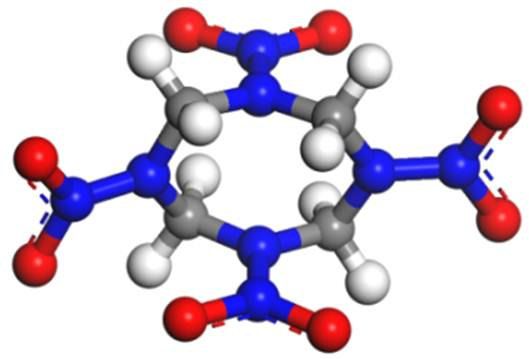Figure 1. Molecular model of HMX.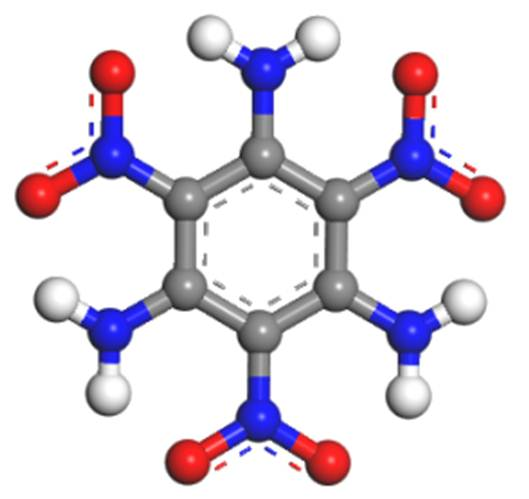Figure 2. Molecular model of TATB.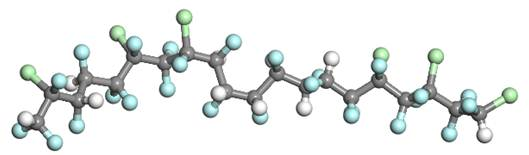Figure 3. Molecular model of F2311.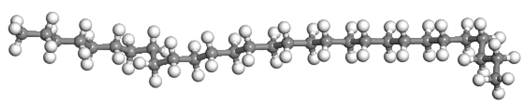Figure 4. Molecular model of PW.

2.2. Crystal Model of JOB-9003 Explosive

Primary simulation cells containing 72 molecules were established in MS for β-HMX, corresponding to 36 (4×3×3) unit cells. Then, the crystal model was put in a box with size a=2.822 nm, b=3.816 nm, c=3.110 nm, α=90°, β=124.3°, γ=90° [2, 17]. Next, 6 HMX molecules in Z axis direction was replaced with TATB molecules. After that, the F2311 and PW which have been optimized were put into the other two crystal surfaces and the initial crystal model of JOB-9003 explosive was got. A total of 2143 atoms were included in JOB-9003 explosive.

After the crystal has been established, MD simulations will be performed in Discover and Forcite modules to optimize the energy and structure of the primary crystal model. Through optimization, the crystal parameters of JOB-9003 explosive is a=2.624 nm, b=3.414 nm, c=2.719 nm, α=90°, β=124.3°, γ=90° and the density of explosive is 1.853 g/cm3. The crystal model of JOB-9003 explosive was shown in Figure. 5.

To investigate the differences among the properties of various crystalline surfaces, the crystal model was cleaved along three crystalline surfaces (1 0 0), (0 1 0), and (0 0 1), respectively to keep its integrality. Then, MD simulations will be conducted at different temperatures and we can get the mechanical properties and binding energy of JOB-9003 explosive in different conditions.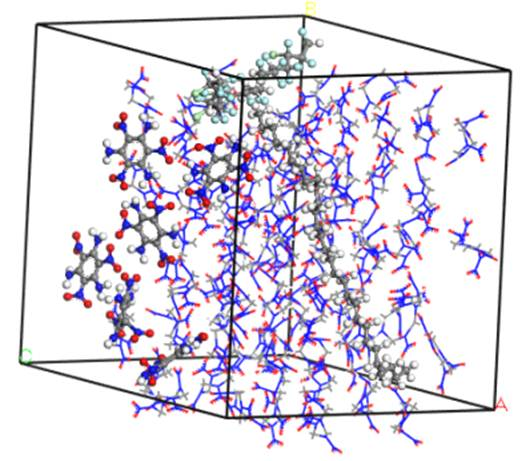Figure 5. Crystal model of JOB-9003 explosive.

2.3. Calculation Conditions

This paper mainly researches the mechanical properties and binding energy of JOB-9003 explosive at different temperatures and investigates the effect of temperature on mechanical properties and binding energy of explosive. The temperature is set as 195 K, 245 K, 295 K, 345 K, 395 K and 445 K, respectively. MD simulations are carried out in the NVT (constant number of particles, volume, and temperature) ensemble with the COMPASS (condensed-phase optimized molecular potentials for atomistic simulation studies) force ﬁeld [18-20]. The step size is 1 fs and the total simulation time is 2×105 fs. The systems are first integrated for 1×105 time steps in all equilibration runs, which are necessary to attain the mechanical and thermal equilibrium, and then followed by the production runs of 1×105 time steps, during which data are collected for subsequent analysis. One frame is saved per 1000 steps, and totally 100 frames are saved to make analysis of static mechanical properties and binding energy.

3. Results Analysis

3.1. Equilibrium of System

To analyze the static mechanical properties of JOB-9003 explosive, the system must reach the equilibrium state. The equilibrium of system is ascertained by the equilibrium in temperature and energy simultaneously; that is to say, when the temperature and energy fluctuate in the range of ±5~10%, the system is believed to have reached the equilibrium state.

Take the (1 0 0) crystal surface as an example, when the temperature is 295 K, the temperature and energy curve versus time is shown in Figure. 6 and Figure. 7, respectively.

What can be seen from Figure. 6 and Figure. 7 is that the temperature and energy increases at the beginning and then both of the temperature and energy fluctuate at a certain range and the system equilibrates in less than 5×104 fs. At last, the temperature fluctuates within ±15 K and the energy fluctuates within ±5~10%, which indicates that the system has reached the equilibrium state. For other conditions, the equilibrium state of the system is based on these two principles.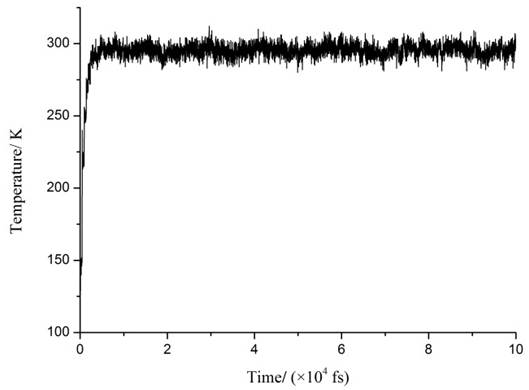Figure 6. Temperature curve versus time.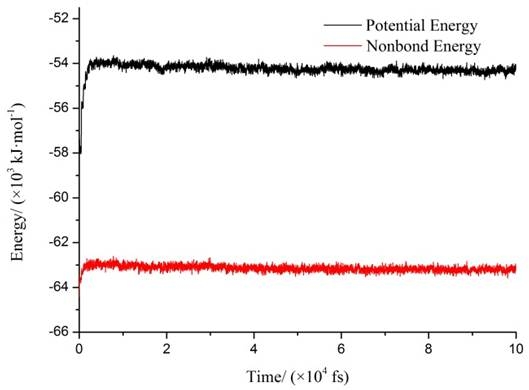Figure 7. Energy curve versus time.

3.2. Mechanical Properties

The relation between stress (σ) and strain (ε) of a material follows the generalized Hooke’s law [21-22] as follows:(1)

Where, Cij (i, j=1, 2, …, 6) is the matrix of elastic coefficients. The matrix is symmetric due to the existence of strain energy, therefore, 21 coefficients are required to describe the relationship between stress and strain for materials.

The mechanical properties for a material mainly conclude tensile modulus (E), bulk modulus (K), shear modulus (G), Poisson’s ratio (γ) and Cauchy pressure (C12C44). Tensile modulus, bulk modulus, and shear modulus can be used to assess the hardness, rigidity or stiffness, tensile strength, fracture strength, and elongation in tension of a material. Besides, mechanical properties can also be used to balance the ability for a material to resist elastic deformation. Meanwhile, the K value can also represent the rupture strength, that is, the greater the value of K is, the more energy will be required for a material to rupture. Cauchy pressure (C12C44) can be used as a criterion to evaluate the brittleness of a material. As a rule, the value of (C12C44) for a ductile material is positive; on the contrary, it is negative for a brittle material. Besides, the more positive the (C12C44) value is, the more ductile the material is. All the mechanical properties can be obtained from the Lamé coefﬁcients (λ and μ) according to the following formulas: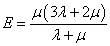(2)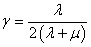(3)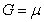(4)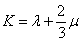(5)

3.2.1. Mechanical Properties of JOB-9003 Explosive at Different Temperatures

When the system reaches the equilibrium state, calculations will be conducted under the Forcite modules in MS and we can get the mechanical properties of explosive. For example, the elastic coefficients and mechanical properties (tensile modulus, Poisson’s ratio, bulk modulus, shear modulus and Cauchy pressure) of JOB-9003 explosive at different temperatures are presented in Table 1.

Table 1. Elastic coefficients and mechanical properties of JOB-9003 explosive at different temperaturesa).

 T (K) 195 245 295 345 395 445 C11 17.198 13.34 15.216 15.067 16.118 16.617 C22 15.088 16.266 16.6 17.358 14.222 18.958 C33 15.69 15.321 13.023 15.451 12.125 11.267 C44 2.672 2.771 2.868 3.162 3.321 3.047 C55 3.829 3.839 4.267 3.458 1.487 3.985 C66 3.759 4.256 3.534 3.982 4.118 2.132 C12 10.084 10.044 9.823 9.48 9.357 8.864 C13 10.23 9.702 10.233 10.268 10.522 9.939 C23 9.587 9.496 8.968 9.173 8.614 9.711 C15 -0.112 -0.726 -0.417 -1.039 -0.113 -1.325 C25 -0.317 0.158 -0.248 -0.454 0.326 -0.259 C35 0.371 0.612 -0.082 -0.179 -0.807 0.161 C46 0.095 -0.021 0.681 0.032 0.455 -0.067 Tensile Modulus (E) 9.468 9.099 8.288 8.184 7.653 5.125 Poisson’s Ratio (γ) 0.368 0.379 0.38 0.37 0.382 0.382 Bulk Modulus (K) 11.917 11.689 11.503 11.396 10.823 7.208 Shear Modulus (G) 3.462 3.32 3.003 2.965 2.768 1.855 Cauchy Pressure (C12－C44) 7.412 7.273 6.955 6.318 6.036 5.817

a) Units for all the elastic coefficients and mechanical properties is GPa except for Poisson’s Ratio.

What can be clearly seen from Table 1 is that the temperature has a direct influence on the elastic coefficients and mechanical properties of JOB-9003 explosive. With the increasing of temperature, the mechanical properties decrease gradually and it is more obvious for the tensile modulus and shear modulus. When the temperature is 195 K, the tensile modulus is 9.468 GPa, the shear modulus is 3.462 GPa; when the temperature is 445 K, the tensile modulus is 5.125 GPa and the shear modulus is 1.855 GPa. The decreasing extent of tensile modulus and shear modulus is 45.87%, 46.42%, respectively. While the decreasing of bulk modulus is 39.51% and it is 21.52% of Cauchy pressure. The decreasing of mechanical properties implies that the rigidity and hardness of the explosive is decreased, i.e., the ability to resist elastic deformation is decreased, while the decreasing of Cauchy pressure indicates that the plastic property is weakened. Based on the MD simulation results, we can conclude that the mechanical properties of explosive is decreased influenced by the temperature, which indicates that the mechanical properties become worse.

3.2.2. Mechanical Properties of Different Crystal Surfaces

The mechanical properties of three crystal surfaces at different temperatures are shown in Table 2.

From Table 2, we can conclude that the mechanical properties of three crystal surfaces decreases obviously with the increasing of temperature, which implies that the mechanical properties would be affected by temperature. The result is consistent with the conclusion drawn above. These variations also illustrates that when subjected to external force, the explosive will deform more easily owing to the decreasing of shear modulus and bulk modulus. Therefore, the temperature has a negative influence on mechanical properties and the effect of temperature on mechanical properties should be taken into consideration in practice. What’s more, to make the explosive keep its favorable mechanical properties, the explosive should be manufactured, stored and transported at a relatively low temperature.

Table 2. Mechanical properties of different crystal surfacesb).

 T (K) 195 245 295 345 395 445 (1 0 0) Tensile Modulus (E) 9.741 9.349 8.393 7.554 6.921 5.386 Poisson’s Ratio (γ) 0.361 0.357 0.362 0.366 0.37 0.365 Bulk Modulus (K) 11.674 10.868 10.136 9.395 8.873 6.649 Shear Modulus (G) 3.579 3.446 3.081 2.765 2.526 1.973 Cauchy Pressure (C12－C44) 5.891 5.723 5.188 5.063 4.717 4.566 (0 1 0) Tensile Modulus (E) 9.566 9.175 8.475 7.321 6.876 5.882 Poisson’s Ratio (γ) 0.365 0.367 0.37 0.372 0.368 0.366 Bulk Modulus (K) 11.810 11.499 10.866 9.532 8.681 7.317 Shear Modulus (G) 3.504 3.356 3.093 2.668 2.513 2.153 Cauchy Pressure (C12－C44) 6.602 6.183 5.193 4.388 4.345 3.777 (0 0 1) Tensile Modulus (E) 9.33 8.906 8.271 7.307 6.081 5.703 Poisson’s Ratio (γ) 0.364 0.368 0.373 0.371 0.372 0.369 Bulk Modulus (K) 11.406 11.245 10.853 9.441 7.918 7.256 Shear Modulus (G) 3.421 3.255 3.012 2.665 2.216 2.083 Cauchy Pressure (C12－C44) 7.237 6.257 5.953 5.47 5.25 4.071

b) Units for all the mechanical properties is GPa except for Poisson’s Ratio.

3.3. Binding Energy

Binding energy (Ebind) between explosive and a polymer is an important criterion for evaluating their miscibility and compatibility. The miscibility of polymer blends plays a crucial role on the mechanical properties of PBXs. Besides, binding energy can well reflect the capacity of the two components blended with each other. Binding energy is deﬁned as the negative value of the intermolecular interaction energy, namely, Ebind=Einter. The intermolecular interaction energy can be evaluated by the total energies of the composite and each component in the equilibrium state. Previous studies [23-25] have shown that binding energy can be used to assess the stability and compatibility of PBXs and the higher the value of binding energy is, the more stable the PBXs is and the better the compatibility between explosive and polymer is.

Binding energy between polymer and explosive can be figured out as follows: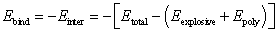(6)

Where, Etotal is the total energy of PBXs, Einter is the intermolecular interaction energy, Eexplosive and Epoly are the total energies of explosive (including HMX, TATB and PW) and fluorine polymer (F2311), respectively.

Based on the MD simulation results, we can get the binding energy between F2311 and JOB-9003 explosive at different temperatures, the results are shown in Figure. 8.Figure 8. Binding energy of JOB-9003 explosive at different temperatures.

From Figure. 8, we can conclude that with the increasing of temperature, the binding energy of the three crystal surfaces decreases monotonously, which implies that the compatibility between F2311 and explosive becomes worse and the stability of explosive decreases. Besides, on the whole, it is obvious that the binding energy between F2311 and different crystalline surfaces changes in the order of (0 0 1) > (1 0 0) > (0 1 0), thus illustrating that the intermolecular interaction energy between F2311 and (0 0 1) crystal surface is stronger and the stability of (0 0 1) crystal surface is better.

4. Conclusions

In this article, we mainly researched the mechanical properties and binding energy of JOB-9003 explosive at different temperatures and investigated the effect of temperature on mechanical properties and stabilities of explosive by molecular dynamics simulation method. The results show that:

(1) With the increasing of temperature, the mechanical properties (tensile modulus, shear modulus, bulk modulus and Cauchy pressure) decrease monotonously, which implies that the toughness, hardness and plastic property of JOB-9003 explosive becomes worse.

(2) The binding energy between F2311 and explosive decreases with the increasing of temperature, which indicates that the compatibility and stability of explosive is weakened. Besides, the binding energy changes in the order of (0 0 1) > (1 0 0) > (0 1 0), thus illustrating that the intermolecular interaction energy between F2311 and (0 0 1) crystal surface is stronger, therefore, the stability of JOB-9003 explosive with F2311 in the (0 0 1) crystal surface is better.

In other words, with the increasing of temperature, both of the mechanical properties and binding energy of explosive decrease, which further implies that the temperature has a negative effect on mechanical properties and thermal stability of explosive. Consequently, the influence of temperature on mechanical properties and stability should be taken into consideration in practice. Besides, the explosive should be manufactured, stored and transported at a relatively low temperature to keep its favorable mechanical properties and stability.

References

1. Discover Accelrys, Materials Studio 3.0, San Diego, CA, 2004.
2. X. F. Ma, F. Zhao, J. J. Xiao, G. F. Ji, W. Zhu, H. M. Xiao, "Simulation study on structure and property of HMX based multi components PBX," Explos. Shock Waves, vol. 27, pp. 109-115, 2007.
3. F. Chen, J. L. Wang, L. Z. Chen, M. L. Duan, Z. X. Wei, B. C. Ren, "Molecular dynamics simulation of mechanical properties and binding energies of ε-CL-20 /F2311 PBXs," J. Atomic Mol. Phys. vol. 32, pp. 360-365, 2015.
4. D. X. Wang, S. S. Chen, L. J. Li, S. H. Jin, "Simulation on the mechanical behavior of the interface structure in ε-HNIW/F2311 PBX," Trans. Beijing Inst. of Tech. vol. 35, pp. 213-217, 2015.
5. D. M. Liu, L. Zhao, J. J. Xiao, J. Chen, G. F. Ji, W. Zhu, F. Zhao, Q. Wu, H. M. Xiao, "Sensitivity criterion and mechanical properties prediction of HMX and RDX crystals at different temperatures- comparative study with molecular dynamics simulation," Chem. J. Chin. Univer. vol. 34, pp. 2558-2565, 2013.
6. Y. Zhou, X. P. Long, X. W. Wei, "Theoretical study on the diffusive transport of 2, 4, 6-trinitrotoluene in polymer-bonded explosive," J. Mol. Model. vol. 17, pp. 3015-3019, 2011.
7. Y. H. Yu, S. S. Chen, X. Li, J. P. Zhu, H. Liang, X. X. Zhang, Q. H. Shu, "Molecular dynamics simulations for 5,5′-bistetrazole-1,1′-diolate (TKX-50) and its PBXs," RSC Adv. vol. 6, pp. 20034-20041, 2016.
8. J. J. Xiao, X. F. Ma, W. Zhu, Y. C. Huang, H. M. Xiao, H. Huang, J. S. Li, "Molecular dynamics simulations of polymer-bonded explosives (PBXs): modeling, mechanical properties and their dependence on temperatures and concentrations of binders," Propellants, Explos., Pyrotech., vol. 32, pp. 355-359, 2007.
9. J. J. Xiao, S. Y. Li, J. Chen, G. F. Ji, W. Zhu, F. Zhao, Q. Wu, H. M. Xiao, "Molecular dynamics study on the correlation between structure and sensitivity for defective RDX crystals and their PBXs," J. Mol. Model. vol. 19, pp. 803-809, 2013.
10. T. Sun, J. J. Xiao, F. Zhao, H. M. Xiao, "Molecular dynamics simulation of compatibility, interface interactions and mechanical properties of CL-20/DNB cocrystal based PBXs," Chin. J. Energ. Mater. vol. 23, pp. 309-314, 2015.
11. J. J. Xiao, L. Zhao, W. Zhu, J. Chen, G. F. Ji, F. Zhao, Q. Wu, H. M. Xiao, "Molecular dynamics study on the relationships of modeling, structural and energy properties with sensitivity for RDX-based PBXs," Sci. China Chem., vol. 55, pp. 2587-2594, 2012.
12. T. Sun, Q. Liu, J. J. Xiao, F. Zhao, H. M. Xiao, "Molecular dynamics simulation of interface interactions and mechanical properties of CL-20/HMX cocrystal and its based PBXs," Acta Chim. Sinica, vol. 72, pp. 1036-1042, 2014.
13. S. Ma, Y. J. Li, Y. Li, Y. J. Luo, "Research on structures, mechanical properties, and mechanical responses of TKX-50 and TKX-50 based PBX with molecular dynamics," J. Mol. Model. vol. 22, pp. 43, 2016.
14. W. Zhu, J. J. Xiao, W. H. Zhu, H. M. Xiao, "Molecular dynamics simulations of RDX and RDX-based plastic-bonded explosives," J. Hazard. Mater. vol. 164, pp. 1082-1088, 2009.
15. S. C. Chang, P. B. Henry, "A study of the crystal structure of β-cyclotetramethylene tetranitramine by neutron diffraction," Acta Cryst. B, vol. 26, pp. 1235-1240, 1970.
16. H. H. Cady, A. C. Larson, D. T. Cromer, "The crystal of α-HMX and a refinement of the structure of β-HMX," Acta Cryst. vol. 16, pp. 617-623, 1963.
17. X. Zhang, Y. L. Wang, "Molecular dynamics simulation of adsorption of mixed gases on JOB-9003 surfaces," Chin. J. Explos. Propellants, vol. 37, pp. 48-52, 2014.
18. H. Sun, "Compass: An ab initio force-field optimized for condense-phase applications- overview with details on alkanes and benzene compounds," J. Phys. Chem. B, vol. 102, pp. 7338-7364, 1998.
19. H. Sun, P. Ren, J. R. Fried, "The COMPASS Force Field: Parameterization and validation for phosphazenes," Comput. Theore. Polym. Sci, vol. 8, pp. 229-246, 1998.
20. S. W. Bunte, H. Sun, "Molecular modeling of energetic materials: the parameterization and validation of nitrate esters in the COMPASS Forcefield," J. Chem. Chem. B, vol. 104, pp. 2477-2489, 2000.
21. J. H. Weiner, "Statistical Mechanics of Elasticity," John Wiley, New York, 1983.
22. J.L. Wu, "Mechanics of Elasticity," Tongji University Press, Shanghai, 1993.
23. L. Qiu, H. M. Xiao, "Molecular dynamics study of binding energies, mechanical properties, and detonation performances of bicyclo-HMX- based PBXs," J. Hazard. Mater. vol. 164, pp. 329-336, 2009.
24. X. J. Xu, J. J. Xiao, H. Huang, J. S. Li, H. M. Xiao, "Molecular dynamics simulations on the structures and properties of ε-CL-20-based PBXs-primary theoretical studies on HEDM formulation design," Sci. China. Ser. B., vol. 50, pp. 737-745, 2007.
25. X. J. Xu, J. J. Xiao, H. Huang, J. S. Li, H. M. Xiao, "Molecular dynamic simulations on the structures and properties of ε-CL-20 (0 0 1)/F2314 PBX," J. Hazard. Mater. vol. 175, pp. 423-428, 2010.

 Contents 1. 2. 2.1. 2.2. 2.3. 3. 3.1. 3.2. 3.3. 4.
Article ToolsAbstractPDF(361K)